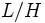# Periodic normal implies amalgam-characteristic

This article gives the statement and possibly, proof, of an implication relation between two subgroup properties. That is, it states that every subgroup satisfying the first subgroup property (i.e., periodic normal subgroup) must also satisfy the second subgroup property (i.e., amalgam-characteristic subgroup)
View all subgroup property implications | View all subgroup property non-implications
Get more facts about periodic normal subgroup|Get more facts about amalgam-characteristic subgroup

## Statement

Suppose$G$ is a group and$H$ is a periodic normal subgroup of$G$: in other words,$H$ is a normal subgroup of$G$ as well as a periodic group (every element of$H$ is of finite order). Then,$H$ is an amalgam-characteristic subgroup of$G$:$H$ is characteristic in$G *_H G$.

## Proof

Given: A group$G$, a periodic normal subgroup$H$ of$G$.$K := G *_H G$.

To prove:$H$ is a characteristic subgroup in$L$.

Proof:

1. By fact (1),$K/H \cong G/H * G/H$.
2.$K/H$ has no nontrivial periodic normal subgroup: By fact (2), if$H$ is a proper subgroup of$G$, then$L/H$ has no nontrivial periodic normal subgroup. If$H = G$, then$L/H$ is trivial and hence has no nontrivial periodic normal subgroup.
3.$H$ is a finite normal subgroup of$K$: Since$H$ is normal in both copies of$G$, it is normal in$K$. Also,$H$ is finite.
4.$H$ is the unique largest periodic normal subgroup of$K$: Suppose$M$ is a finite normal subgroup of$K$. Then, by fact (4), the image of$M$ in the quotient map$K \to K/H$ is a normal subgroup of$K/H$. Also, since$M$ is periodic, its image is periodic. In step (2), we concluded that$K/H$ has no nontrivial periodic normal subgroup. Thus, the image of$M$ is trivial, so$M \le H$.
5.$H$ is characteristic in$K$: This follows since it is the unique largest periodic normal subgroup of$K$.